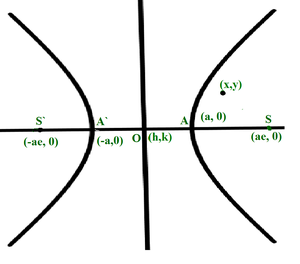# Check if a point is inside, outside or on a Hyperbola

• Difficulty Level : Medium
• Last Updated : 25 Nov, 2021

Given a hyperbola centered at (h, k), with semi-major axis a, semi-minor axis b, both aligned with the Cartesian plane, the task is to determine if the point (x, y) lies within the area bounded by the hyperbola or not.Examples:

Input: h = 0, k = 0, x = 2, y = 1, a = 4, b = 5
Output: Inside

Input: h = 1, k = 2, x = 200, y = 100, a = 6, b = 5
Output: Outside

Approach: The given problem can be solved by solving the equation of hyperbola mentioned below, for the given point (x, y):Based on the evaluated value of the above equation, the output of the program is as follows:

• Less than 1: The point lies inside the hyperbola.
• Equal to 1: The point lies on the hyperbola
• Greater than 1: The point lies outside the hyperbola.

Below is the implementation of the above approach:

## C++

 // C++ program for the// above approach#include using namespace std; // Function to check if the point// (x, y) lies inside, on or// outside the given hyperbolavoid checkpoint(int h, int k, int x,                int y, int a, int b){     // Stores the value of the equation    int p = (pow((x - h), 2) / pow(a, 2))            - (pow((y - k), 2) / pow(b, 2));     // Generate output based on value of p    if (p > 1) {        cout << "Outside";    }    else if (p == 1) {        cout << "On the Hyperbola";    }    else {        cout << "Inside";    }} // Driver Codeint main(){    int h = 0, k = 0, x = 2;    int y = 1, a = 4, b = 5;     checkpoint(h, k, x, y, a, b);     return 0;}

## Java

 // Java program for the above approachimport java.io.*;import java.lang.*;import java.util.*; class GFG{ // Function to check if the point// (x, y) lies inside, on or// outside the given hyperbolastatic void checkpoint(int h, int k, int x,                       int y, int a, int b){         // Stores the value of the equation    int p = (int)(Math.pow((x - h), 2) / Math.pow(a, 2)) -            (int)(Math.pow((y - k), 2) / Math.pow(b, 2));     // Generate output based on value of p    if (p > 1)    {        System.out.println("Outside");    }    else if (p == 1)    {        System.out.println("On the Hyperbola");    }    else    {        System.out.println("Inside");    }} // Driver Codepublic static void main(String[] args){    int h = 0, k = 0, x = 2;    int y = 1, a = 4, b = 5;     checkpoint(h, k, x, y, a, b);}} // This code is contributed by Kingash

## Python3

 # Python3 program for the above approachfrom math import pow # Function to check if the point# (x, y) lies inside, on or# outside the given hyperboladef checkpoint(h, k, x, y, a, b):     # Stores the value of the equation    p = ((pow((x - h), 2) // pow(a, 2)) -         (pow((y - k), 2) // pow(b, 2)))     # Generate output based on value of p    if (p > 1):        print("Outside")         elif (p == 1):        print("On the Hyperbola");         else:        print("Inside") # Driver Codeif __name__ == "__main__":     h = 0    k = 0    x = 2    y = 1    a = 4    b = 5     checkpoint(h, k, x, y, a, b) # This code is contributed by AnkThon

## C#

 // C# program for the above approachusing System; class GFG{ // Function to check if the point// (x, y) lies inside, on or// outside the given hyperbolastatic void checkpoint(int h, int k, int x,                       int y, int a, int b){         // Stores the value of the equation    int p = (int)(Math.Pow((x - h), 2) / Math.Pow(a, 2)) -            (int)(Math.Pow((y - k), 2) / Math.Pow(b, 2));     // Generate output based on value of p    if (p > 1)    {        Console.WriteLine("Outside");    }    else if (p == 1)    {        Console.WriteLine("On the Hyperbola");    }    else    {        Console.WriteLine("Inside");    }} // Driver Codepublic static void Main(string[] args){    int h = 0, k = 0, x = 2;    int y = 1, a = 4, b = 5;     checkpoint(h, k, x, y, a, b);}} // This code is contributed by ukasp

## Javascript

 

Output:

Inside

Time Complexity: O(1)
Auxiliary Space: O(1)

My Personal Notes arrow_drop_up